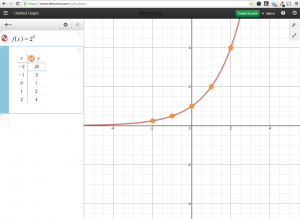# f(x)=2^x

f(x)=2^x 2014-09-15T19:34:25+00:00graph of f(x) = 2^x including points plotted